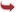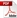International Journal of Scientific and Research Publications

#### IJSRP, Volume 6, Issue 11, November 2016 Edition [ISSN 2250-3153]A Mixed Type Approximation Method for Radiation and Scattering Problems
J. Sriranganesan, H. M. Nasir
Abstract: We consider the scattering problem given by the Exterior Helmholtz equation in two dimension by the finite element method. We use a finite element approximation method for the matrix element which correspond to the exact non-local radiation boundary condition on the artificial boundary. We propose a mixed type approximation method to implement the exact Dirichlet to Neumann (DtN) boundary condition that directly gives an approximation matrix for the Helmholtz problem. We use non- uniform partitioning of the artificial boundary by considering some example for which analytical solution was computed. We prove the convergence of the solution of our method to the exact solution when the exact DtN boundary condition is applied on a circular boundary.
[VIEW FULL PAPER][DOWNLOAD]

Reference this Research Paper (copy & paste below code):

J. Sriranganesan, H. M. Nasir (2018); A Mixed Type Approximation Method for Radiation and Scattering Problems; Int J Sci Res Publ 6(11) (ISSN: 2250-3153). http://www.ijsrp.org/research-paper-1116.php?rp=P596016